Chapman Electric Supply, Inc. is now  part of Eckart Supply!  learn more>>

# How to Calculate Underground Pull Box Sizing

Determining the size of underground pull and junction boxes to meet NEC 314.16 code standards is required to prevent damage to conductor insulation. Calculating the correct size of pull box needed can be complicated, so please feel free to contact us or call 800-767-1576 if you need assistance.

NEC 314.28 code requirements apply to installations with conductors of 4 AWG or larger that are required to be insulated, and for cables containing conductors of 4 AWG or larger.  These apply to splices, straight pulls, U pulls and angle pulls.

## Calculating Pull Box Enclosure Sizes:

Minimum size requirements:  Minimum distance from where conductors enter to the opposite wall cannot be less than eight times the trade size of the largest raceway.

### Straight Pulls

Straight pulls are when the conduit enters the box on one side, and leaves the box on the opposite side, as seen in the diagram below. Straight pulls need 8 times the the diameter of the largest conduit/raceway.

In this case, simply multiply your largest raceway size by 8, and that will give you the minimum length for your box.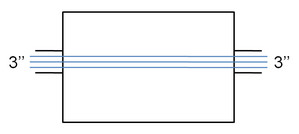Largest Raceway Size x 8 = Minimum Box Length

For example, if  your raceway is 3'':

3'' x 8 = 24''

### Splices, Angle Pulls, U Pulls

Angle Pulls are when conduit enters the box, makes a 90 degree turn and then leaves the box on the adjacent wall. U Pulls consist of the conduit entering and leaving the same side of the box.

These calculations also start with the largest raceway size, but then get a little more complicated. You need to multiply your largest raceway by 6, then add the sizes of any other raceways on the same wall and row to get minimum box size.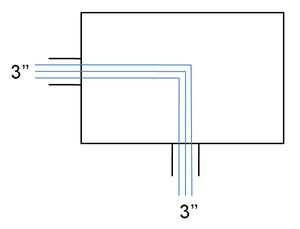(Largest Raceway Size x 6) + Sizes of Raceways on the Same Row = Minimum Box Length

For example, if you have a single Splice, Angle or U pull of 3'' raceway:

3'' x 6 = 18''

### Multiple Raceways

If you have multiple rows of raceways, calculate each one separately and choose the largest distance.

If you a have multiple rows of raceways, use the largest size calculated.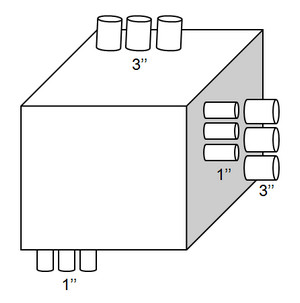For example, if you have a row with 3-3'' raceways, and another row with 3-1'' raceways:

Calculate Row 1: (3'' x 6) + 3'' + 3'' = 24''

Calculate Row 2: (1'' x 6) + 1'' + 1'' = 8''

Choose 24'', the larger of the 2 sizes.

### Distance Between Raceways

Additionally, the distance between raceways that enclose the same conductor cannot be less than six times the largest raceway, measured from the raceways’ nearest edge-to-nearest edge.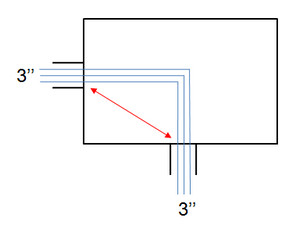Largest Raceway Size x 6 = Minimum Distance Between Raceways

For example, if you have a 3'' raceway from left to bottom:

3'' x 6 = 18''

### Multiple Types of Pulls

Multiple types of pulls can be done within the same box, for example, a straight pull with an angle pull in the same box.

If you a have multiple types of pulls, you must calculate for each of the different types of pulls, using the correct formulas, then choose largest of the sizes calculated.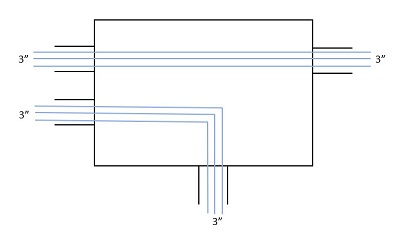For example, if you have a box with a straight pull of 3'' raceway, and an angle pull with a 3'' raceway:

Calculate the straight pull, left to right: 3'' x 8 = 24''

Calculate the angle pull, left to right: (3'' x 6) + 3" = 21''

Choose 24'', the larger of the 2 sizes for your left to right calculation.

Calculate the angle pull, top to bottom: 3'' x 6 = 18''

Your box must be at least 24" wide and 18" tall.

## Pull Box Calculation Steps:

You will want to calculate both horizontal (left to right and right to left) and vertical (top to bottom and bottom to top) dimensions, as well as calculating the distance between raceways. Because this can be confusing, you will want to draw out the configuration.

For example, if you have a pull box with 1-3'' raceway on the left, 1-2'' raceway on the left  1-2'' raceway on the right, and 1-3'' raceway on the bottom.  So you have a 2'' straight pull and a 3'' angle pull.

STEP 1:
Determine the size of each raceway you will be using.
STEP 2:
Determine if you need a Straight or Splice/Angle/U Pull, as you need to use different formulas.
STEP 3:
Calculate the Horizontal Dimension: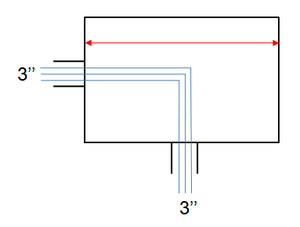• Straight Pull:
• Left to Right: 8'' x 2 = 16''
• Right to Left: 8'' x 2 = 16''
• Your pull box must be at least 16” wide.
• Angle Pull:
• Left to Right: (3'' x 6) + 2'' = 20''
• Right to Left:  None

STEP 4:
Calculate the Vertical Dimension: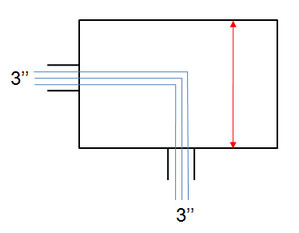1. Angle Pull (Angle/Splice/U Pulls Only)
• Top to Bottom: None
• Bottom to Top: 3'' x 6 = 18''

STEP 5:

Calculate the Distance Between Raceways (Angle/Splice/U Pulls Only)
1. Distance between Raceways: 3'' x 6 = 18''

STEP 6:

Compare the distance between raceways vs. Vertical and Horizontal Distances and choose the larger of the 2. (Angle/Splice/U Pulls Only)
1. (Distance between Raceways = 18”) = (Vertical Distance = 18”)
2. (Distance between Raceways = 18”) < (Horizontal Distance = 120”)
3. Your pull box must be at least 20'' wide by 18'' tall.STEP 7: Determine Sizes if Multiple Rows of Raceways (skip this step if you have a single raceway) and choose the largest size calculated.

For example, if you have a row with 3-3'' raceways, and another row with 3-1'' raceways:

1. Calculate Row 1: (3'' x 6) + 3'' + 3'' = 24''
2. Calculate Row 2: (1'' x 6) + 1'' + 1'' = 8''
3. Choose 24'', the larger of the 2 sizes.

Need help choosing the right size pull boxes? Our knowledgeable staff would be happy to assist you. Contact us or call 800-767-1576.

### Other Articles You May Enjoy:

Choosing the Right Underground Enclosures

Determining Proper ANSI Load Ratings for Underground Pull Boxes